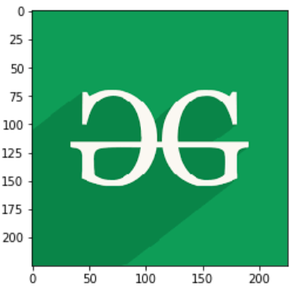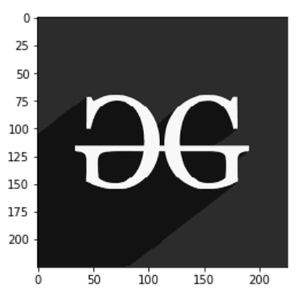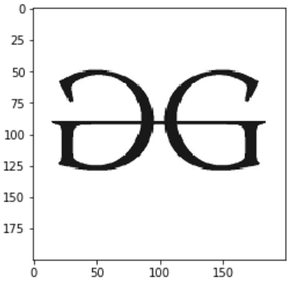# How to Display an Image in Grayscale in Matplotlib?

• Difficulty Level : Easy
• Last Updated : 01 Feb, 2022

In this article, we are going to depict images using matplotlib module in grayscale representation i.e. image representation using two colors only i.e. black and white.

### Required modules

• PIL is the Python Imaging Library which provides the python interpreter with image editing capabilities. The Image module provides a class with the same name which is used to represent a PIL image. The module also provides a number of factory functions, including functions to load images from files, and to create new images. PIL.Image.open() method in PIL module opens and identifies the given image file.
• Matplotlib is a plotting library for creating static, animated, and interactive visualizations in Python. The matplotlib module can be used in Python scripts, the Python and IPython shell, web application servers, and various graphical user interface toolkits like Tkinter, awxPython, etc.

Step-by-step Approach:

• Import required modules

## Python3

 `# importing libraries.``import` `numpy as np``import` `matplotlib.pyplot as plt``from` `PIL ``import` `Image`

• Displaying Original picture.

## Python3

 `# storing image path``fname ``=` `r``'g4g.png'` `# opening image using pil``image ``=` `Image.``open``(fname)` `# plottingimage``plt.imshow(image)``plt.show()`

Output:• Displaying Grayscale image, store the image path here let’s say it fname. Now open the image using PIL image method and convert it to L mode If you have an L mode image, that means it is a single-channel image – normally interpreted as grayscale. It only stores a grayscale, not color. Plotting the image as cmap = ‘gray’ convert the colours. All the work is done you can now see your image.

## Python3

 `# storing image path``fname ``=` `r``'g4g.png'` `# opening image using pil``image ``=` `Image.``open``(fname).convert(``"L"``)` `# maping image to gray scale``plt.imshow(image, cmap``=``'gray'``)``plt.show()`

Output:Below are some programs which depict how to display an image in grayscale using Matplotlib module:

Example 1:

Image used:## Python3

 `# importing libraries.``import` `numpy as np``import` `matplotlib.pyplot as plt``from` `PIL ``import` `Image`  `# storing image path``fname ``=` `r``'gfg.png'` `# opening image using pil``image ``=` `Image.``open``(fname).convert(``"L"``)` `# mapping image to gray scale``plt.imshow(image, cmap``=``'gray'``)``plt.show()`

Output:Example 2:

Image Used:## Python3

 `# importing libraries.``import` `numpy as np``import` `matplotlib.pyplot as plt``from` `PIL ``import` `Image`  `# storing image path``fname ``=` `r``'geeks.png'` `# opening image using pil``image ``=` `Image.``open``(fname).convert(``"L"``)` `# mapping image to gray scale``plt.imshow(image, cmap``=``'gray'``)``plt.show()`

Output:My Personal Notes arrow_drop_up